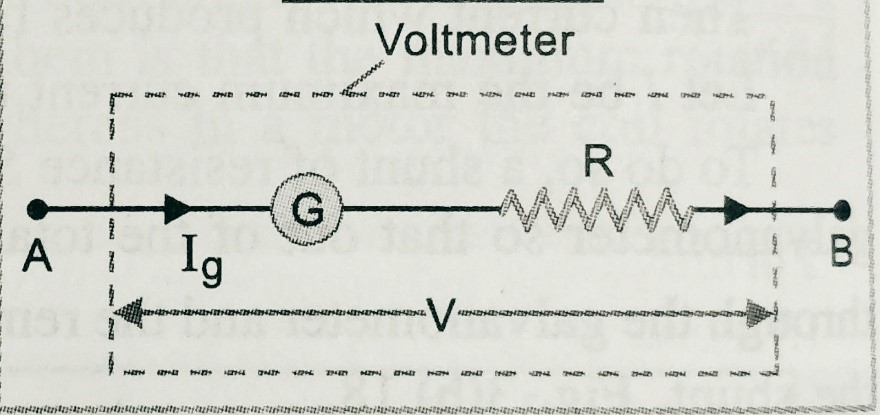Categories

# Conversion of galvanometer into voltmeter

In this topic we will discuss about the ‘ Conversion of galvanometer into voltmeter ‘ . What is ammeter? and how can we convert a Galvanometer into voltmeter?

Before to read this topic ‘ Conversion of galvanometer into voltmeter ‘  students must know about the galvanometer and shunt .

### This topic is very important topic  for class 12th board examination and for other competitive examination ( for medical and engineering entrance examinations )

Voltmeter-

It is high resistance galvanometer used to measure potential difference between two points of a conductor or circuit.

A galvanometer can be converted into voltmeter by connecting high value resistance in series with the galvanometer( as shown in figure).Let, G is the resistance of the galvanometer,

IG  is the current passes through the galvanometer,

R is the high value resistance  in series with the galvanometer.

Let,

V is the potential difference of the galvanometer.

So we can write V = IG ( R + G)

Hence  R =( V/IG)- G ;

Resistance of the voltmeter Rs = R + G ;

So we can say ,for ideal voltmeter the resistance of the voltmeter should be infinity.

### This topic is very important for all state  class 12th board examination and for other entrance examination , therefore it is important to solve the assignment of this topic .

In the assignment , we provide all kind of questions like , objective , short answer type, long answer type , numericals and hots . After solving the assignment of this chapter students will be able to improve there knowledge on this chapter.

To get the complete assignment of magnetic effect of current click here-

But students must solve the questions base on its previous topic.

We are providing the link , so that students should clear all topic of this chapter- click here for ammeter